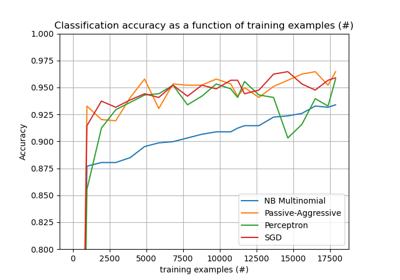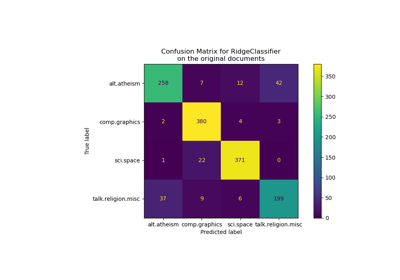# sklearn.linear_model.PassiveAggressiveClassifier¶

class sklearn.linear_model.PassiveAggressiveClassifier(*, C=1.0, fit_intercept=True, max_iter=1000, tol=0.001, early_stopping=False, validation_fraction=0.1, n_iter_no_change=5, shuffle=True, verbose=0, loss='hinge', n_jobs=None, random_state=None, warm_start=False, class_weight=None, average=False)[source]

Passive Aggressive Classifier

Read more in the User Guide.

Parameters
Cfloat, default=1.0

Maximum step size (regularization). Defaults to 1.0.

fit_interceptbool, default=True

Whether the intercept should be estimated or not. If False, the data is assumed to be already centered.

max_iterint, default=1000

The maximum number of passes over the training data (aka epochs). It only impacts the behavior in the fit method, and not the partial_fit method.

New in version 0.19.

tolfloat or None, default=1e-3

The stopping criterion. If it is not None, the iterations will stop when (loss > previous_loss - tol).

New in version 0.19.

early_stoppingbool, default=False

Whether to use early stopping to terminate training when validation. score is not improving. If set to True, it will automatically set aside a stratified fraction of training data as validation and terminate training when validation score is not improving by at least tol for n_iter_no_change consecutive epochs.

New in version 0.20.

validation_fractionfloat, default=0.1

The proportion of training data to set aside as validation set for early stopping. Must be between 0 and 1. Only used if early_stopping is True.

New in version 0.20.

n_iter_no_changeint, default=5

Number of iterations with no improvement to wait before early stopping.

New in version 0.20.

shufflebool, default=True

Whether or not the training data should be shuffled after each epoch.

verboseinteger, default=0

The verbosity level

lossstring, default=”hinge”

The loss function to be used: hinge: equivalent to PA-I in the reference paper. squared_hinge: equivalent to PA-II in the reference paper.

n_jobsint or None, default=None

The number of CPUs to use to do the OVA (One Versus All, for multi-class problems) computation. None means 1 unless in a joblib.parallel_backend context. -1 means using all processors. See Glossary for more details.

random_stateint, RandomState instance, default=None

Used to shuffle the training data, when shuffle is set to True. Pass an int for reproducible output across multiple function calls. See Glossary.

warm_startbool, default=False

When set to True, reuse the solution of the previous call to fit as initialization, otherwise, just erase the previous solution. See the Glossary.

Repeatedly calling fit or partial_fit when warm_start is True can result in a different solution than when calling fit a single time because of the way the data is shuffled.

class_weightdict, {class_label: weight} or “balanced” or None, default=None

Preset for the class_weight fit parameter.

Weights associated with classes. If not given, all classes are supposed to have weight one.

The “balanced” mode uses the values of y to automatically adjust weights inversely proportional to class frequencies in the input data as n_samples / (n_classes * np.bincount(y))

New in version 0.17: parameter class_weight to automatically weight samples.

averagebool or int, default=False

When set to True, computes the averaged SGD weights and stores the result in the coef_ attribute. If set to an int greater than 1, averaging will begin once the total number of samples seen reaches average. So average=10 will begin averaging after seeing 10 samples.

New in version 0.19: parameter average to use weights averaging in SGD

Attributes
coef_array, shape = [1, n_features] if n_classes == 2 else [n_classes, n_features]

Weights assigned to the features.

intercept_array, shape =  if n_classes == 2 else [n_classes]

Constants in decision function.

n_features_in_int

Number of features seen during fit.

New in version 0.24.

n_iter_int

The actual number of iterations to reach the stopping criterion. For multiclass fits, it is the maximum over every binary fit.

classes_array of shape (n_classes,)

The unique classes labels.

t_int

Number of weight updates performed during training. Same as (n_iter_ * n_samples).

loss_function_callable

Loss function used by the algorithm.

References

Online Passive-Aggressive Algorithms <http://jmlr.csail.mit.edu/papers/volume7/crammer06a/crammer06a.pdf> K. Crammer, O. Dekel, J. Keshat, S. Shalev-Shwartz, Y. Singer - JMLR (2006)

Examples

>>> from sklearn.linear_model import PassiveAggressiveClassifier
>>> from sklearn.datasets import make_classification

>>> X, y = make_classification(n_features=4, random_state=0)
>>> clf = PassiveAggressiveClassifier(max_iter=1000, random_state=0,
... tol=1e-3)
>>> clf.fit(X, y)
PassiveAggressiveClassifier(random_state=0)
>>> print(clf.coef_)
[[0.26642044 0.45070924 0.67251877 0.64185414]]
>>> print(clf.intercept_)
[1.84127814]
>>> print(clf.predict([[0, 0, 0, 0]]))



Methods

 Predict confidence scores for samples. Convert coefficient matrix to dense array format. fit(X, y[, coef_init, intercept_init]) Fit linear model with Passive Aggressive algorithm. get_params([deep]) Get parameters for this estimator. partial_fit(X, y[, classes]) Fit linear model with Passive Aggressive algorithm. Predict class labels for samples in X. score(X, y[, sample_weight]) Return the mean accuracy on the given test data and labels. set_params(**kwargs) Set and validate the parameters of estimator. Convert coefficient matrix to sparse format.
decision_function(X)[source]

Predict confidence scores for samples.

The confidence score for a sample is proportional to the signed distance of that sample to the hyperplane.

Parameters
Xarray-like or sparse matrix, shape (n_samples, n_features)

Samples.

Returns
array, shape=(n_samples,) if n_classes == 2 else (n_samples, n_classes)

Confidence scores per (sample, class) combination. In the binary case, confidence score for self.classes_ where >0 means this class would be predicted.

densify()[source]

Convert coefficient matrix to dense array format.

Converts the coef_ member (back) to a numpy.ndarray. This is the default format of coef_ and is required for fitting, so calling this method is only required on models that have previously been sparsified; otherwise, it is a no-op.

Returns
self

Fitted estimator.

fit(X, y, coef_init=None, intercept_init=None)[source]

Fit linear model with Passive Aggressive algorithm.

Parameters
X{array-like, sparse matrix} of shape (n_samples, n_features)

Training data

ynumpy array of shape [n_samples]

Target values

coef_initarray, shape = [n_classes,n_features]

The initial coefficients to warm-start the optimization.

intercept_initarray, shape = [n_classes]

The initial intercept to warm-start the optimization.

Returns
selfreturns an instance of self.
get_params(deep=True)[source]

Get parameters for this estimator.

Parameters
deepbool, default=True

If True, will return the parameters for this estimator and contained subobjects that are estimators.

Returns
paramsdict

Parameter names mapped to their values.

partial_fit(X, y, classes=None)[source]

Fit linear model with Passive Aggressive algorithm.

Parameters
X{array-like, sparse matrix} of shape (n_samples, n_features)

Subset of the training data

ynumpy array of shape [n_samples]

Subset of the target values

classesarray, shape = [n_classes]

Classes across all calls to partial_fit. Can be obtained by via np.unique(y_all), where y_all is the target vector of the entire dataset. This argument is required for the first call to partial_fit and can be omitted in the subsequent calls. Note that y doesn’t need to contain all labels in classes.

Returns
selfreturns an instance of self.
predict(X)[source]

Predict class labels for samples in X.

Parameters
Xarray-like or sparse matrix, shape (n_samples, n_features)

Samples.

Returns
Carray, shape [n_samples]

Predicted class label per sample.

score(X, y, sample_weight=None)[source]

Return the mean accuracy on the given test data and labels.

In multi-label classification, this is the subset accuracy which is a harsh metric since you require for each sample that each label set be correctly predicted.

Parameters
Xarray-like of shape (n_samples, n_features)

Test samples.

yarray-like of shape (n_samples,) or (n_samples, n_outputs)

True labels for X.

sample_weightarray-like of shape (n_samples,), default=None

Sample weights.

Returns
scorefloat

Mean accuracy of self.predict(X) wrt. y.

set_params(**kwargs)[source]

Set and validate the parameters of estimator.

Parameters
**kwargsdict

Estimator parameters.

Returns
selfobject

Estimator instance.

sparsify()[source]

Convert coefficient matrix to sparse format.

Converts the coef_ member to a scipy.sparse matrix, which for L1-regularized models can be much more memory- and storage-efficient than the usual numpy.ndarray representation.

The intercept_ member is not converted.

Returns
self

Fitted estimator.

Notes

For non-sparse models, i.e. when there are not many zeros in coef_, this may actually increase memory usage, so use this method with care. A rule of thumb is that the number of zero elements, which can be computed with (coef_ == 0).sum(), must be more than 50% for this to provide significant benefits.

After calling this method, further fitting with the partial_fit method (if any) will not work until you call densify.

## Examples using sklearn.linear_model.PassiveAggressiveClassifier¶Out-of-core classification of text documents¶Comparing various online solvers¶Classification of text documents using sparse features¶